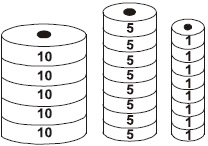# User Forum

Subject :IMO    Class : Class 2

What is the total value of the given disks ?A96
B97
C98
D99

Number of disks with value 10 = 5Total value of disks with value 10 = 5 x 10 = 50Number of disks with value 5 = 8Total value of disks with value 5 = 8 x 5 = 40Number of disks with value 1 = 8Total value of disks with value 1 = 8 x 1 = 8Total value of the disks = 50 40 8 = 98